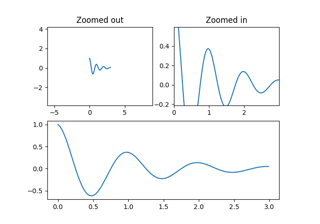# matplotlib.pyplot.subplot¶

matplotlib.pyplot.subplot(*args, **kwargs)[source]

Add an Axes to the current figure or retrieve an existing Axes.

This is a wrapper of `Figure.add_subplot` which provides additional behavior when working with the implicit API (see the notes section).

Call signatures:

```subplot(nrows, ncols, index, **kwargs)
subplot(pos, **kwargs)
subplot(**kwargs)
subplot(ax)
```
Parameters
*argsint, (int, int, index), or `SubplotSpec`, default: (1, 1, 1)

The position of the subplot described by one of

• Three integers (nrows, ncols, index). The subplot will take the index position on a grid with nrows rows and ncols columns. index starts at 1 in the upper left corner and increases to the right. index can also be a two-tuple specifying the (first, last) indices (1-based, and including last) of the subplot, e.g., `fig.add_subplot(3, 1, (1, 2))` makes a subplot that spans the upper 2/3 of the figure.

• A 3-digit integer. The digits are interpreted as if given separately as three single-digit integers, i.e. `fig.add_subplot(235)` is the same as `fig.add_subplot(2, 3, 5)`. Note that this can only be used if there are no more than 9 subplots.

projection{None, 'aitoff', 'hammer', 'lambert', 'mollweide', 'polar', 'rectilinear', str}, optional

The projection type of the subplot (`Axes`). str is the name of a custom projection, see `projections`. The default None results in a 'rectilinear' projection.

polarbool, default: False

If True, equivalent to projection='polar'.

sharex, sharey`Axes`, optional

Share the x or y `axis` with sharex and/or sharey. The axis will have the same limits, ticks, and scale as the axis of the shared axes.

labelstr

A label for the returned axes.

Returns
`axes.SubplotBase`, or another subclass of `Axes`

The axes of the subplot. The returned axes base class depends on the projection used. It is `Axes` if rectilinear projection is used and `projections.polar.PolarAxes` if polar projection is used. The returned axes is then a subplot subclass of the base class.

Other Parameters
**kwargs

This method also takes the keyword arguments for the returned axes base class; except for the figure argument. The keyword arguments for the rectilinear base class `Axes` can be found in the following table but there might also be other keyword arguments if another projection is used.

Property

Description

`adjustable`

{'box', 'datalim'}

`agg_filter`

a filter function, which takes a (m, n, 3) float array and a dpi value, and returns a (m, n, 3) array

`alpha`

scalar or None

`anchor`

(float, float) or {'C', 'SW', 'S', 'SE', 'E', 'NE', ...}

`animated`

bool

`aspect`

{'auto', 'equal'} or float

`autoscale_on`

bool

`autoscalex_on`

bool

`autoscaley_on`

bool

`axes_locator`

Callable[[Axes, Renderer], Bbox]

`axisbelow`

bool or 'line'

`box_aspect`

float or None

`clip_box`

`Bbox`

`clip_on`

bool

`clip_path`

Patch or (Path, Transform) or None

`facecolor` or fc

color

`figure`

`Figure`

`frame_on`

bool

`gid`

str

`in_layout`

bool

`label`

object

`navigate`

bool

`navigate_mode`

unknown

`path_effects`

`AbstractPathEffect`

`picker`

None or bool or float or callable

`position`

[left, bottom, width, height] or `Bbox`

`prop_cycle`

unknown

`rasterization_zorder`

float or None

`rasterized`

bool

`sketch_params`

(scale: float, length: float, randomness: float)

`snap`

bool or None

`title`

str

`transform`

`Transform`

`url`

str

`visible`

bool

`xbound`

unknown

`xlabel`

str

`xlim`

(bottom: float, top: float)

`xmargin`

float greater than -0.5

`xscale`

{"linear", "log", "symlog", "logit", ...} or `ScaleBase`

`xticklabels`

unknown

`xticks`

unknown

`ybound`

unknown

`ylabel`

str

`ylim`

(bottom: float, top: float)

`ymargin`

float greater than -0.5

`yscale`

{"linear", "log", "symlog", "logit", ...} or `ScaleBase`

`yticklabels`

unknown

`yticks`

unknown

`zorder`

float

Notes

Creating a new Axes will delete any pre-existing Axes that overlaps with it beyond sharing a boundary:

```import matplotlib.pyplot as plt
# plot a line, implicitly creating a subplot(111)
plt.plot([1, 2, 3])
# now create a subplot which represents the top plot of a grid
# with 2 rows and 1 column. Since this subplot will overlap the
# first, the plot (and its axes) previously created, will be removed
plt.subplot(211)
```

If you do not want this behavior, use the `Figure.add_subplot` method or the `pyplot.axes` function instead.

If no kwargs are passed and there exists an Axes in the location specified by args then that Axes will be returned rather than a new Axes being created.

If kwargs are passed and there exists an Axes in the location specified by args, the projection type is the same, and the kwargs match with the existing Axes, then the existing Axes is returned. Otherwise a new Axes is created with the specified parameters. We save a reference to the kwargs which we use for this comparison. If any of the values in kwargs are mutable we will not detect the case where they are mutated. In these cases we suggest using `Figure.add_subplot` and the explicit Axes API rather than the implicit pyplot API.

Examples

```plt.subplot(221)

# equivalent but more general
ax1 = plt.subplot(2, 2, 1)

# add a subplot with no frame
ax2 = plt.subplot(222, frameon=False)

# add a polar subplot
plt.subplot(223, projection='polar')

# add a red subplot that shares the x-axis with ax1
plt.subplot(224, sharex=ax1, facecolor='red')

# delete ax2 from the figure
plt.delaxes(ax2)

# add ax2 to the figure again
plt.subplot(ax2)

# make the first axes "current" again
plt.subplot(221)
```

## Examples using `matplotlib.pyplot.subplot`¶Controlling view limits using margins and sticky_edges¶